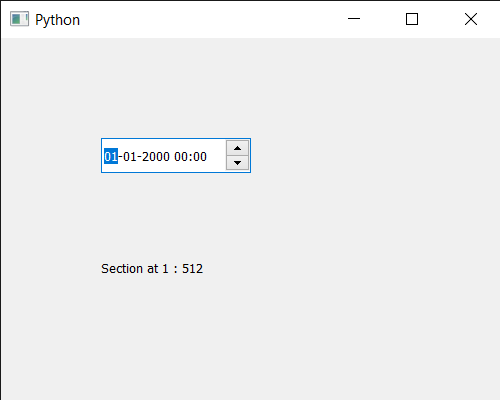Skip to content
Related Articles
PyQt5 QDateTimeEdit – Finding Section at given index
• Last Updated : 14 Jul, 2020

In this article we will see how we can find the section at the given index in the QDateTimeEdit widget. There are many sections in the QDateTimeedit widget like, year, month, hour, minute section. So sometimes, we there is a need to get the section of the specific index.

In order to do this we will use `sectionAt` method with the QDateTimeEdit object.

Syntax : datetimeedit.sectionAt(n)

Argument : It takes integer as argument

Return : It returns section object but when printed it shows the corresponding value of it.

Below is the implementation

 `# importing libraries``from` `PyQt5.QtWidgets ``import` `*` `from` `PyQt5 ``import` `QtCore, QtGui``from` `PyQt5.QtGui ``import` `*` `from` `PyQt5.QtCore ``import` `*` `import` `sys`` ` ` ` `class` `Window(QMainWindow):`` ` `    ``def` `__init__(``self``):``        ``super``().__init__()`` ` `        ``# setting title``        ``self``.setWindowTitle(``"Python "``)`` ` `        ``# setting geometry``        ``self``.setGeometry(``100``, ``100``, ``500``, ``400``)`` ` `        ``# calling method``        ``self``.UiComponents()`` ` `        ``# showing all the widgets``        ``self``.show()`` ` `    ``# method for components``    ``def` `UiComponents(``self``):`` ` `        ``# creating a QDateTimeEdit widget``        ``datetimeedit ``=` `QDateTimeEdit(``self``)`` ` `        ``# setting geometry``        ``datetimeedit.setGeometry(``100``, ``100``, ``150``, ``35``)`` ` ` ` `        ``# creating a label``        ``label ``=` `QLabel(``"GeeksforGeeks"``, ``self``)`` ` `        ``# setting geometry to the label``        ``label.setGeometry(``100``, ``200``, ``200``, ``60``)`` ` `        ``# making label multi line``        ``label.setWordWrap(``True``)`` ` `        ``# getting section at index 1``        ``value ``=` `datetimeedit.sectionAt(``1``)`` ` `        ``# setting text to the label``        ``label.setText(``"Section at 1 : "` `+` `str``(value))`` ` ` ` ` ` ` ` `# create pyqt5 app``App ``=` `QApplication(sys.argv)`` ` `# create the instance of our Window``window ``=` `Window()`` ` `# start the app``sys.exit(App.``exec``())`

Output :Attention geek! Strengthen your foundations with the Python Programming Foundation Course and learn the basics.

To begin with, your interview preparations Enhance your Data Structures concepts with the Python DS Course. And to begin with your Machine Learning Journey, join the Machine Learning – Basic Level Course

My Personal Notes arrow_drop_up